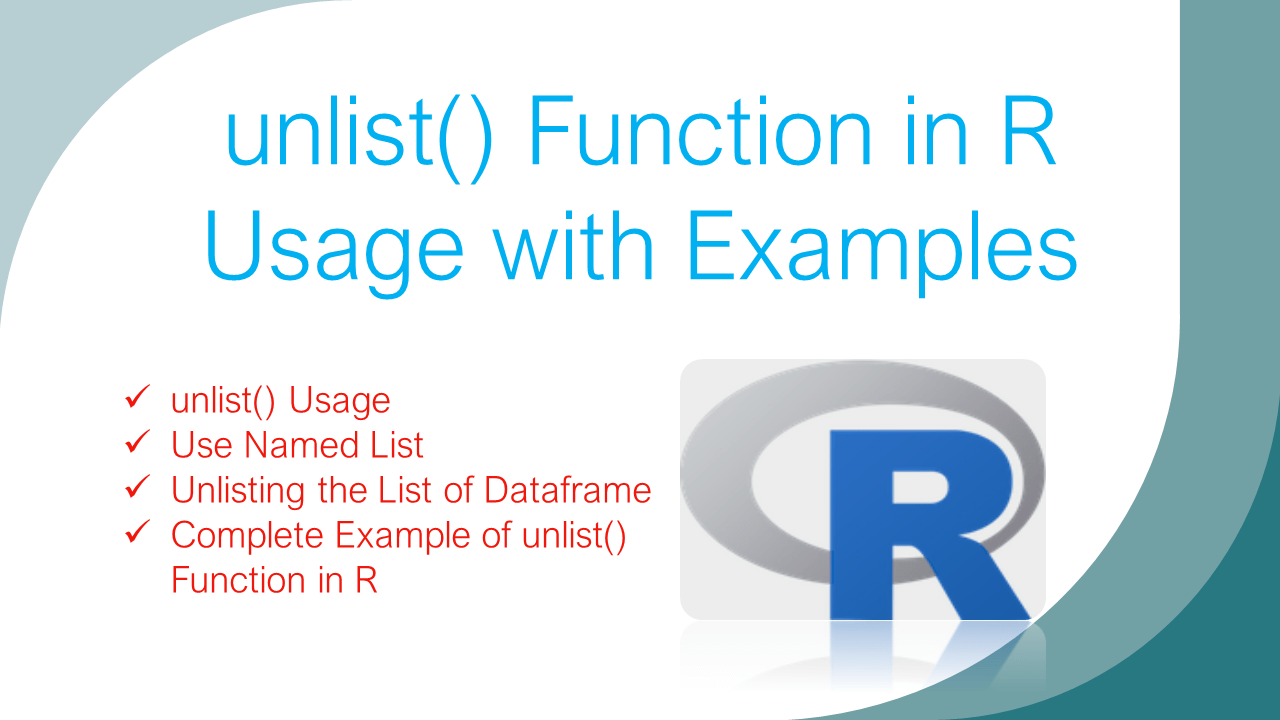# unlist() Function in R – Usage with Examples

unlist() function in R takes a list as an argument and returns a vector. A list in R contains heterogeneous elements meaning can contain elements of different types whereas a vector in R is a basic data structure containing elements of the same data type.

A list can hold characters, numeric, and complex types like data.frame, vector matric e.t.c. To unlist these elements from the list to vector use unlist() function.

## 1. Syntax of unlist() Function

``````
# Syntax of unlist()
unlist(x, recursive = TRUE, use.names = TRUE)
``````

### 1.1. Parameters of unlist()

Following are the parameters of unlist() function.

• x – Input R object which you wanted to unlist. It can be a list or vector.
• recursive – Recursively apply unlisting elements of x. Accept values TRUE/FALSE.
• use.names – Use FALE to ignore the names in the vector. Accept values TRUE/FALSE.

### 1.2. unlist() Return value of unlist()

unlist() function returns a vector with the elements from the list. When used use.names=FALSE it returns a vector without names.

## 2. R unlist() Usage

Use unlist() function to convert a list to a vector by unlisting the elements from a list. A list in R contains heterogeneous elements meaning can contain elements of different types whereas a vector in R is a basic data structure containing elements of the same data type.

Let’s convert the simple list. first, create a list by using the list() function.

``````
# Create list
li <- list('A','B','C')
print(li)

# unlist() usage
v <- unlist(li)
print(v)
``````

Yields below output.

## 3. Use Named List

Most of the time when we have to use a list in R, we will use a nested list with names, also called a named list. Let’s use the named list on unlist() function and validate the result. Here. I have created a list object with vector values.

``````
# Use unlist() on Named list
li <- list(col1 = c(10, 20, 30),
col2 = c(1, 40, 3),
col3 = c(30, 20))
print(li)

v <- unlist(li)
print(v)
``````

Yields below output. Note that using a named list, creates a vector with names.

If you don’t want names you can ignore them by using use.names=FALSE.

## 4. Unlisting the List of Dataframe

As I explained in the beginning, a list in R can contain the data frame hence let’s create a list with a dataframe element and convert it to a vector. While unlisting, if you want to return the dataframe column names, you can specify the use.names=TRUE. If you don’t want the column names, you can specify the use.names=FALSE. The following example uses the default option which is use.names=TRUE.

Here, I have a list with a dataframe containing 3 columns col1,col2, and col3.

``````
# Convert List with data.frame
li <- list(data.frame(col1 = c(10, 20, 30),
col2 = c(1, 40, 3),
col3 = c(30, 20,4)) )

print(li)
v <- unlist(li,use.names=FALSE)
print(v)
``````

Yields below output.

## 5. Complete Example of unlist() Function in R

Following is a complete example of using unlist() function.

``````
# Create list
li <- list('A','B','C')
print(li)

# unlist() usage
v <- unlist(li)
print(v)

# Create Named list
li <- list(col1 = c(10, 20, 30),
col2 = c(1, 40, 3),
col3 = c(30, 20))
print(li)

# Use unlist() on Named list
v <- unlist(li)
print(v)
``````

## 6. Conclusion

In this article, you have learned unlist() function in R? unlist function syntax, and usage with different parameters. Finally learned how to change a list into a vector using this function with examples. You can find the complete example from this article at Github R Programming Examples Project.

## References

### Naveen (NNK)

Naveen (NNK) is a Data Engineer with 20+ years of experience in transforming data into actionable insights. Over the years, He has honed his expertise in designing, implementing, and maintaining data pipelines with frameworks like Apache Spark, PySpark, Pandas, R, Hive and Machine Learning. Naveen journey in the field of data engineering has been a continuous learning, innovation, and a strong commitment to data integrity. In this blog, he shares his experiences with the data as he come across. Follow Naveen @ @ LinkedIn